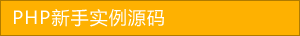﻿ pdo操作数据库入门教程 - php高级应用 - PHP粉丝网

# pdo操作数据库入门教程

##### 发布:smiling　来源: PHP粉丝网 　添加日期：2014-09-11 10:19:18　浏览: 评论：0

1. \$dsn = "mysql:host=localhost;dbname=test"
2. \$db = new PDO(\$dsn'root''');
3. \$count = \$db-＞exec("INSERT INTO foo SET name = 'heiyeluren',gender='男',time=NOW()");
4. echo \$count
5. \$db = null;

\$db = new PDO(\$dsn, 'root', '');

\$count = \$db-＞exec("INSERT INTO foo SET name = 'heiyeluren',gender='男',time=NOW()");
echo \$count;

\$db = new PDO(\$dsn, 'root', '', array(PDO::ATTR_PERSISTENT =＞ true));

1. foreach(\$db-＞query("SELECT * FROM foo")){
2. 　print_r(\$row);

1. \$rs = \$db-＞query("SELECT * FROM foo");
2. while(\$row = \$rs-＞fetch()){
3. 　print_r(\$row);

1. \$rs = \$db-＞query("SELECT * FROM foo");
2. \$result_arr = \$rs-＞fetchAll();
3. print_r(\$result_arr);
4.
5.
6. Array
7. ( =＞ Array(
8. 　[id] =＞ 1
9. 　 =＞ 1
10. 　[name] =＞ heiyeluren
11. 　 =＞ heiyeluren
12. 　[gender] =＞ 男
13. 　 =＞ 男
14. 　[time] =＞ 2006-10-28 23:14:23
15. 　 =＞ 2006-10-28 23:14:23

1. \$db-＞setAttribute(PDO::ATTR_CASE, PDO::CASE_UPPER);
2. \$rs = \$db-＞query("SELECT * FROM foo");
3. \$rs-＞setFetchMode(PDO::FETCH_ASSOC);
4. \$result_arr = \$rs-＞fetchAll();
5. print_r(\$result_arr);

PDO::CASE_LOWER -- 强制列名是小写

PDO::CASE_NATURAL -- 列名按照原始的方式

PDO::CASE_UPPER -- 强制列名为大写

PDO::FETCH_ASSOC -- 关联数组形式

PDO::FETCH_NUM -- 数字索引数组形式

PDO::FETCH_BOTH -- 两者数组形式都有,这是缺省的

PDO::FETCH_OBJ -- 按照对象的形式,类似于以前的 mysql_fetch_object()

1. \$rs = \$db-＞prepare("SELECT * FROM foo");
2. \$rs-＞execute();
3. while(\$row = \$rs-＞fetch()){
4. 　print_r(\$row);

1. \$rs = \$db-＞query("SELECT COUNT(*) FROM foo");
2. \$col = \$rs-＞fetchColumn();
3. echo \$col

1. 面向对象的方式

1. try {
2. 　\$db = new PDO('mysql:host=localhost;dbname=test'\$user\$pass);
3. 　\$db = null;
4. } catch (PDOException \$e) {
5. 　print "Error: " . \$e-＞getMessage() . "＜br/＞"
6. 　die();

PDOException异常类的属性结构:

1. class PDOException extends Exception
2. 　public \$errorInfo = null; // 错误信息，可以调用 PDO::errorInfo() 或 PDOStatement::errorInfo()来访问
3. //开源代码phpfensi.com
4. 　protected \$message// 异常信息，可以试用 Exception::getMessage() 来访问
5. 　protected \$code// SQL状态错误代码，可以使用 Exception::getCode() 来访问

1. class Exception
2. 　// 属性
3. 　protected \$message = 'Unknown exception'// 异常信息
4. 　protected \$code = 0; // 用户自定义异常代码
5. 　protected \$file// 发生异常的文件名
6. 　protected \$line// 发生异常的代码行号
7.
8. 　// 方法
9. 　final function getMessage(); // 返回异常信息
10. 　final function getCode(); // 返回异常代码
11. 　final function getFile(); // 返回发生异常的文件名
12. 　final function getLine(); // 返回发生异常的代码行号
13. 　final function getTrace(); // backtrace() 数组
14. 　final function getTraceAsString(); // 已格成化成字符串的 getTrace() 信息

2. 使用面向过程的方法,先看代码:

1. \$db = new PDO('mysql:host=localhost;dbname=test'\$user\$pass);
2. \$rs = \$db-＞query("SELECT aa,bb,cc FROM foo");
3. if (\$db-＞errorCode() != '00000'){
4. 　print_r(\$db-＞errorInfo());
5. 　exit
6. \$arr = \$rs-＞fetchAll();
7. print_r(\$arr);
8. \$db = null;

PDO和PDOStatement对象有errorCode() 和 errorInfo() 方法,如果没有任何错误,errorCode() 返回的是:00000,否则就会返回一些错误代码,errorInfo() 返回的一个数组,包括PHP定义的错误代码和MySQL的错误代码和错误信息,数组结构如下:

1. Array
2. 　 =＞ 42S22
3. 　 =＞ 1054
4. 　 =＞ Unknown column 'aaa' in 'field list'

## 相关文章